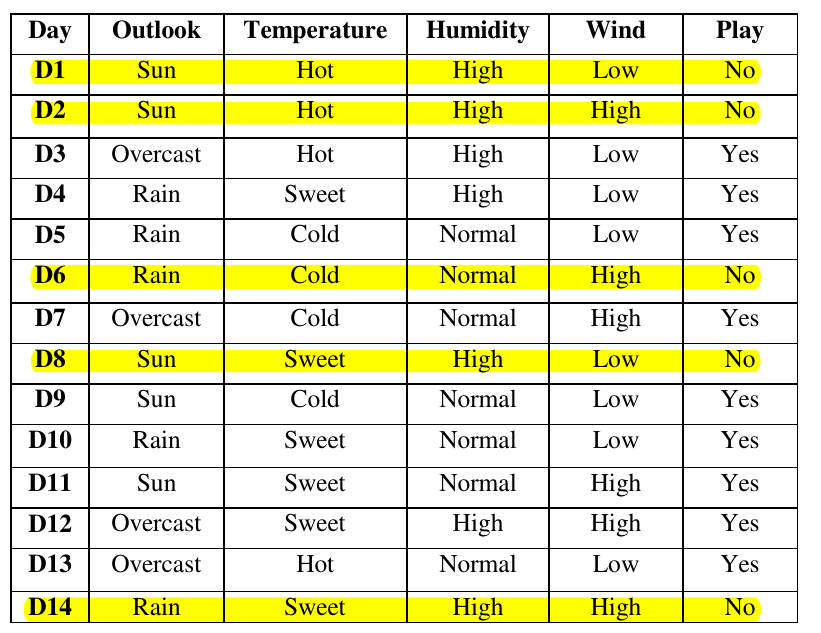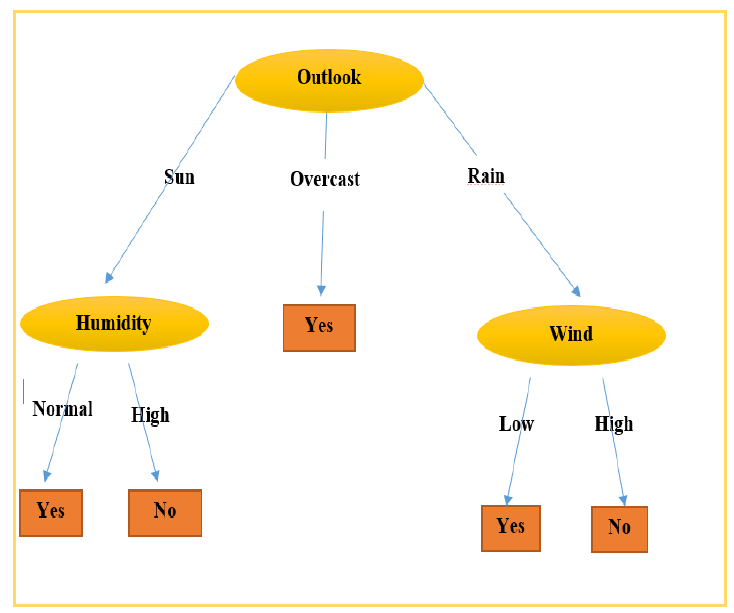# 【面试考】【入门】决策树算法ID3，C4.5和CART

## ID3• 因为整个数据集只有两个类别，他们的分布概率分别是$$\frac{9}{14}$$$$\frac{5}{14}$$，所以根据Entropy是：$$Entropy(S)=-(\frac{9}{14}*log_2(\frac{9}{14})+\frac{5}{14}*log_2(\frac{5}{14}))=0.94$$

$$Entropy(S_{sun})=-(\frac{2}{5}*log_2(\frac{2}{5})+\frac{3}{5}*log_2(\frac{3}{5}))=0.971$$
$$Entropy(S_{overcast})=-(\frac{4}{4}*log_2(\frac{4}{4})+\frac{0}{4}*log_2(\frac{0}{4}))=0$$
$$Entropy(S_{rain})=-(\frac{3}{5}*log_2(\frac{3}{5})+\frac{2}{5}*log_2(\frac{2}{5}))=0.971$$

$$IG(S,Outlook)=Entropy(S)-(\frac{5}{14}*Entropy(S_{sun})+\frac{5}{14}*Entropy(S_{overcast})+\frac{5}{14}*Entropy(S_{rain}))=0.246$$

$$IG(S,Wind)=0.048$$
$$IG(S,Temperature)=0.0289$$
$$IG(S,Humidity)=0.1515$$### ID3的缺点

1. 如果样本中存在一个特征，这个特征中所有值都不相同（比方说是连续值的特征），这样可以想想的出假设用这个特征作为划分，那么信息增益一定是非常大的，因为所有的划分中都只会包含一个样本；对于具有很多值的属性它是非常敏感的，例如，如果我们数据集中的某个属性值对不同的样本基本上是不相同的，甚至更极端点，对于每个样本都是唯一的，如果我们用这个属性来划分数据集，它会得到很大的信息增益，但是，这样的结果并不是我们想要的。
2. ID3不能处理连续值属性；
3. ID3算法不能处理具有缺失值的样本；
4. 非常容易过拟合。

## C4.5

$IV(S,a)=-\sum_{v\in values(a)}{\frac{|x\in S|value(x,a)=v|}{|S|}*log_2(\frac{|x\in S|value(x,a)=v|}{|S|})}$

• S就是数据集样本，$$|S|$$就是样本数量；
• a是某一个特征，比方说Outlook或者是Wind,然后$$v\in values(a)$$就是v就是a这个特征中的某一个值；
• $$|x\in S|value(x,a)=v|$$这个就是某一个特征a是v的样本数量；
然后决策树之前使用信息增益Information Gain来作为分裂特征的选择，现在使用增益率IG rate：

$IGR(S,a)=\frac{IG(S,a)}{IV(S,a)}$

C4.5如何处理缺失值

• 如果是训练数据中出现了缺失数据，那么就会考虑这个缺失数据所有可能的值。比方说一开始的数据库中，D1的Outlook变成了缺失值，那么D1的Outlook就会有$$\frac{4}{13}$$的概率是Sun，有$$\frac{4}{13}$$的概率是Overcast，有$$\frac{5}{13}$$的概率是Rain，然后其实也可以理解为这个样本就会变成3个样本，这三个样本有着不同的权重。
• 如果是在预测数据中出现了缺失数据，那么同样的，认为这个数据的这个缺失数据可能是任何可能的值，这个概率就是看决策树中Outlook划分的子集的样本数量。这个地方可能有点难懂，不理解的可以看这个博文：
机器学习笔记（7）——C4.5决策树中的缺失值处理

C4.5对决策树的剪枝处理

• 预剪枝：从上到下进行剪枝，如果精度没有提升，那么就剪掉，这个处理在训练模型的过程中进行；
• 后剪枝：从下到上进行剪枝，如果剪掉精度可以提升，就剪掉，这个处理过程是在模型训练结束之后再进行的。
通常来说后者会比前者保留更多的分支，欠拟合的风险小，但是训练时间的开销会大一些。
更具体地内容推荐这篇博文，讲的清晰易懂(没有必要看懂这个博文中的Python实现过程，毕竟现在sklearn库中都封装好了)：
机器学习笔记（6）——C4.5决策树中的剪枝处理和Python实现